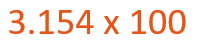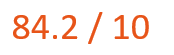Smartick is an advanced online program that teaches kids math and coding in only 15 min. a day
Try it for free!

Apr17

# Multiplying and Dividing Decimal Numbers by Powers of 10

In this post, we will learn how to multiply and divide decimal numbers by 10, 100, 1000 … It is as simple as moving the decimal point of the decimal number to the left or right.

### Multiplying decimal numbers by 10, 100, 1000 …

To multiply a decimal number by 10, 100, 1000 … all you have to do is move the decimal point to the right of the decimal as many positions as the number of zeros.

For example:Since the 100 has two zeros, we will move the decimal point two places to the right. Therefore, the result is 315.4

### Dividing decimal numbers by 10, 100, 1000 …

To divide a decimal number by 10, 100, 1000 … all you have to do is move the decimal point to the left as many positions as the number of zeros.

For example:Since 10 has one zero we will move the decimal point one position to the left. Therefore, the result is 8.42

Check out these posts if you want more practice with multiplying and dividing decimal numbers:

Remember that you can register on Smartick and learn many elementary math topics! Sign up for free.

Learn More:

Latest posts by Smartick (see all)